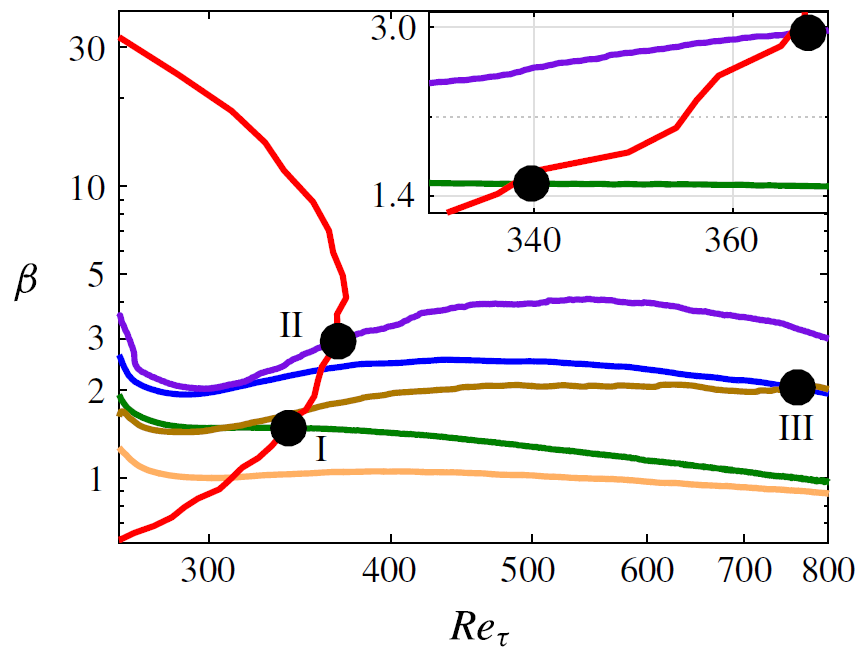# Well-resolved LES of pressure-gradient turbulent boundary layers

The databases on this page are publicly available. Please refer to the original publications when using the data.

Contact Ricardo Vinuesa (rvinuesa@mech.kth.se) if you have any questions about the data.

### LES of APG TBLs up to Retheta=4,000 and beta=4Clauser pressure-gradient parameter beta as a function of friction Reynolds number for the 5 flat-plate cases shared here. Figure extracted from Bobke et al., J. Fluid Mech. 820 (2017).

Database

- The database is described in: A. Bobke, R. Vinuesa, R. Orlu and P. Schlatter. History effects and near equilibrium in adverse-pressure-gradient turbulent boundary layers. J. Fluid Mech., 820, 667-692 (2017).

- The local edge velocity (Ue) and 99% boundary-layer thickness (delta99) were calculated using the method in:

R. Vinuesa, A. Bobke, R. Orlu and P. Schlatter. On determining characteristic length scales in pressure-gradient turbulent boundary layers. Phys. Fluids, 28, 055101 (2016).

- The data is stored in .mat format here.

- The two cases with constant beta are b1n and b2n.

- The three cases with constant power-law exponent are m13n, m16n and m18n.

- The data is organized into Matlab structures, where each entry is a streamwise position.

- The database includes mean flow, Reynolds-stress tensor, integral quantities and turbulent kinetic energy budget terms.